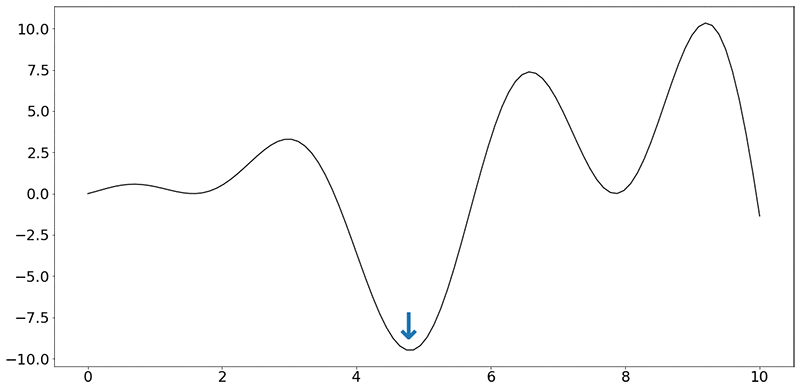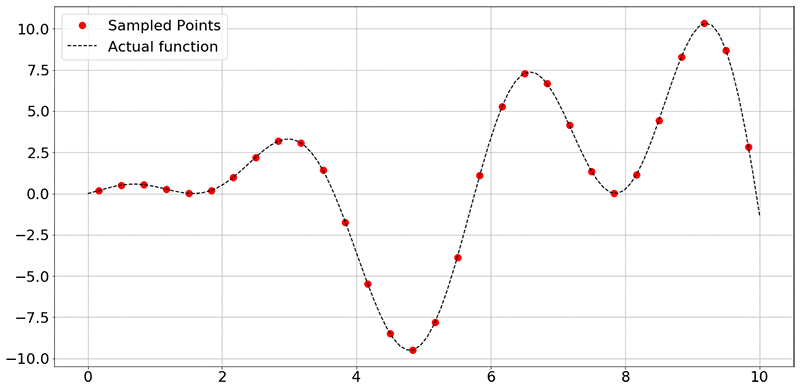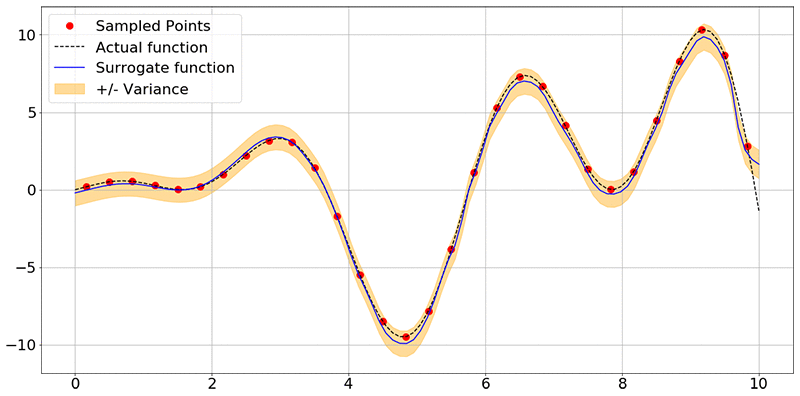Blog

5000+ Members

MEETUPS

LEARN, CONNECT, SHARE

JOB POSTINGS

INDEED POSTINGS

Browse through the latest deep learning, ai, machine learning postings from Indeed for the GTA.

CONTACT

CONNECT WITH US

Are you looking to sponsor space, be a speaker, or volunteer, feel free to give us a shout.

Building a deep neural net–based surrogate function for global optimization using PyTorch on Amazon SageMaker

Optimization is the process of finding the minimum (or maximum) of a function that depends on some inputs, called design variables. Customer X has the following problem: They are about to release a new car model to be designed for maximum fuel efficiency. In reality, thousands of parameters that represent tuning parameters relating to the engine, transmission, suspension, and so on. The combinations result in varying fuel efficiency values.

However, for this post, assume that they want to measure this efficiency as the gallons of fuel burned per hour when traveling at a particular speed, all other parameters being constant. Therefore, the “function” to be minimized is “gallons of fuel burned per hour” and the design variable is “speed.” This one-dimensional optimization problem asks the question: “What speed should the car be driven at for burning the minimum amount of fuel per hour,” which is a greatly simplified version of the thousands of actual parameters to be considered.

Assume that the objective function (f) looks like the following synthetic function:

f(x) = x⋅sin(x)+x⋅cos(2x)Ignoring the units on the x and y axes, your task is to find the minimum of this function, indicated by the blue arrow. Even when dealing with a single dimension, it is impractical to run the car over every speed value (speed being a real number).

For this post, you have a budget of running 30 experiments, each “experiment” consisting of running the car on a test rig at that speed, measuring and collecting the average value of fuel burned per hour. This gives you 30 values of fuel burned, corresponding to 30 values of speeds, and nothing more. There is also no guarantee that there was an experiment conducted at the value of speed indicated by the minimum (blue arrow in the figure).

Each experiment can actually take hours to set up. Because it is impractical to do more than a certain number of such experiments, this type of function is called an expensive, black-box function. It’s expensive because the function takes time to return a value, and black-box as the experiments conducted can’t be written as mathematical expressions.

The entire field of optimization research is targeted towards creating algorithms to solve these kinds of problems. In this post, you use a neural network to approximate the function (f) above. This trained approximation of the function, also known as a “surrogate model,” can be used instead of actual experiments! If the trained model is a good approximation of the actual function, the model can be used to predict the fuel burned (output) for any value of speed (input).

Technical approach

For a sample Jupyter notebook that walks through all these steps, see Build a Deep Neural Global Optimizer.

Given the function (f), measure the value of the output given the values of various inputs. You create a simple, four-layer network, based on the recommendations in Scalable Bayesian Optimization Using Deep Neural Networks:

1. Input layer (tanh activation)
2. Hidden layer 1 (tanh activation)
3. Hidden layer 2 (tanh activation)
4. Output layer (ReLU activation)

In PyTorch, this can be written as follows:

def __init__(self, D_in, H, D, D_out):
"""
In the constructor, instantiate two nn.Linear modules and assign them as
member variables.
"""
super(Net, self).__init__()
self.inputlayer = nn.Linear(D_in, H)
self.middle = nn.Linear(H, H)
self.lasthiddenlayer = nn.Linear(H, D)
self.outputlayer = nn.Linear(D, D_out)

Where D_in, H, D and D_out are used to define the parameter matrix sizes within the function.

You are also required to specify the activation function for each neuron, and how inputs are transformed in the forward pass:

def forward(self, x):
"""
In the forward function, accept a variable of input data and return
a variable of output data. Use modules defined in the constructor, as
well as arbitrary operators on variables.
"""
y_pred = self.outputlayer(self.PHI(x))
return y_pred

def PHI(self, x):
h_relu = self.inputlayer(x).tanh()
for i in range(2):
h_relu = self.middle(h_relu).tanh()
phi = self.lasthiddenlayer(h_relu)
return phi

In the train function, use Mean Squared Error as the loss function and use the Adam optimizer:

self.network = Net(features, self.H, self.D, 1) # here we suppose that D_out = 1
loss_fn = torch.nn.MSELoss(size_average=True)

To collect data from the experiments, sample the function f(x) = x⋅sin(x)+x⋅cos(2x) at random points. In the figure below, the black dashed line represents all values of f(x) in that range of x (here, 0 to 10), and the red dots represent the 30 sampled points.

To reiterate, the goal of the network is to use the training data (x and y axis values corresponding to the sampled data points) to learn to approximate the function. Provided that the neural network has learned a good approximation of the original function f(x), you can use the trained model to predict the values of the outputs, given inputs, without running an expensive or a time-consuming experiment.If you’re interested in the more technical details, see Scalable Bayesian Optimization Using Deep Neural Networks. In brief, a Bayesian linear regressor is added to the last hidden layer of a deep neural network. This results in adaptive basis regression, a well-established statistical technique that scales linearly in the number of observations. These “basis functions” are parameterized using the weights and biases of the deep neural network. Finally, the mean and variance of the prediction can then be calculated using the formulae (4) and (5) in the Scalable Bayesian Optimization paper. So, you are not only obtaining a function approximation, but also the uncertainty associated with the predicted points.

Given the small size of the input vector, you train the model on a notebook instance with the conda36_pytorch kernel. I highly encourage you to resort to distributed training using Amazon SageMaker rather than local training when appropriate. The following command starts the training process:

deepgaussian.train(DOE,yvalues)

In PyTorch, the training loop is implemented as follows:

for t in range(self.num_epochs):
y_pred = self.network(self.X)
loss = loss_fn(y_pred.view(-1), self.Y.view(-1))
loss.backward()
optimizer.step()

Obtain the following output indicating that the network has been trained:

Optimization terminated successfully.
Current function value: 6.652170
Iterations: 49
Function evaluations: 99

Finally, plot the surrogate model using a set of test values (xtest), as follows:

mean, var = deepgaussian.predict(x_test)
plt.figure(figsize=(20,10))
plt.rcParams.update({'font.size': 22})
plt.plot(DOE, yvalues, "ro",label='Sampled Points',markersize=10)
plt.plot(xtest[:,0], fvals, "k--", label = 'Actual function')
plt.plot(xtest[:,0], mean, "blue",label='Surrogate function')
plt.fill_between(xtest[:, 0], mean + np.sqrt(var), mean - np.sqrt(var), color="orange", alpha=0.4, label='+/- Variance')
plt.grid()
plt.legend()
plt.show()

You obtain the following image:As you can see, the network has learned the shape of the function f(x) accurately, and also associates some uncertainty with each point it used for prediction. Here, the blue lines are prediction means and the orange band is the uncertainty associated with each of the predictions.

Conclusion

At this point, the model can be used to predict any number of experimental output values within a confidence interval, without actually performing the experiment. What is more useful is using an optimization package to find the optimum input value that corresponds to the minimum f(x) value. To start, see the scipy.optimize or inspyred packages.

Lastly, this is a starter example that runs locally on a notebook instance. Get started now by launching the Amazon SageMaker console and exploring distributed training on Amazon Sagemaker. For large-scale optimization jobs, consider doing distributed training on Amazon SageMaker by submitting the PyTorch script to the Amazon SageMaker Pytorch estimator.Shreyas Subramanian is a AI/ML specialist Solutions Architect, and helps customers by using Machine Learning to solve their business challenges using the AWS platform.

Days
:
Hours
:
Minutes
:
Seconds

Plug yourself into AI and don't miss a beat

Toronto AI is a social and collaborative hub to unite AI innovators of Toronto and surrounding areas. We explore AI technologies in digital art and music, healthcare, marketing, fintech, vr, robotics and more. Toronto AI was founded by Dave MacDonald and Patrick O'Mara.